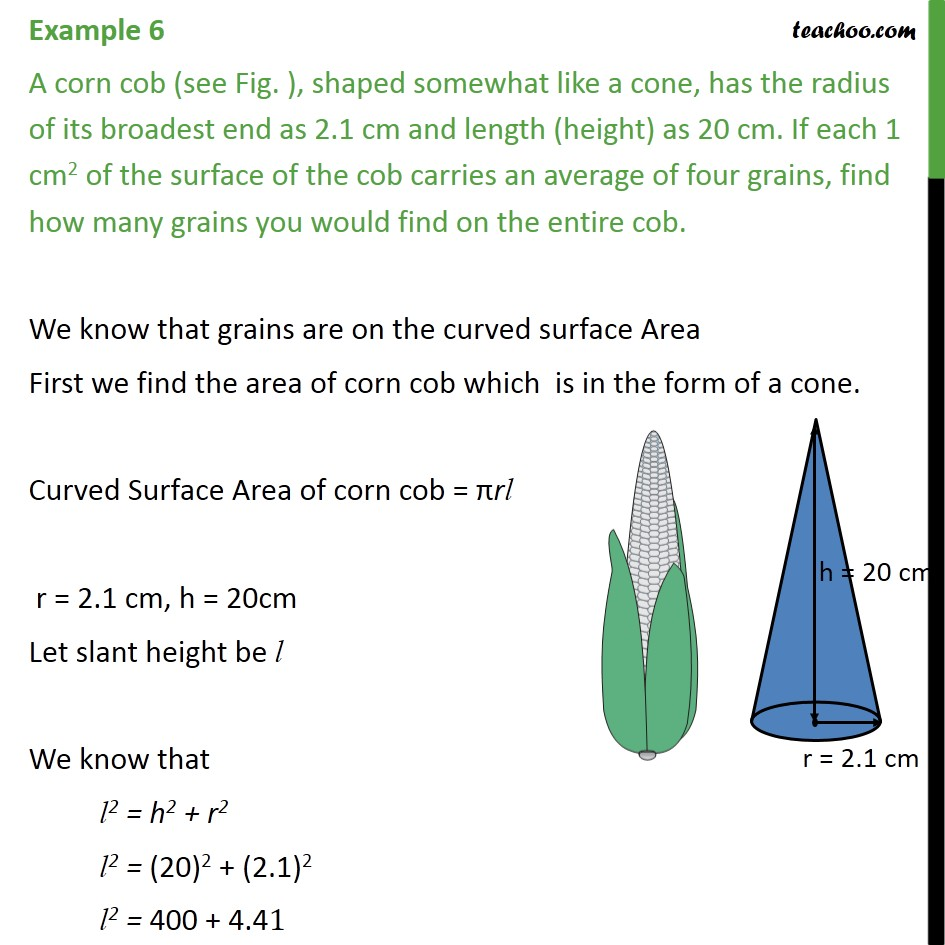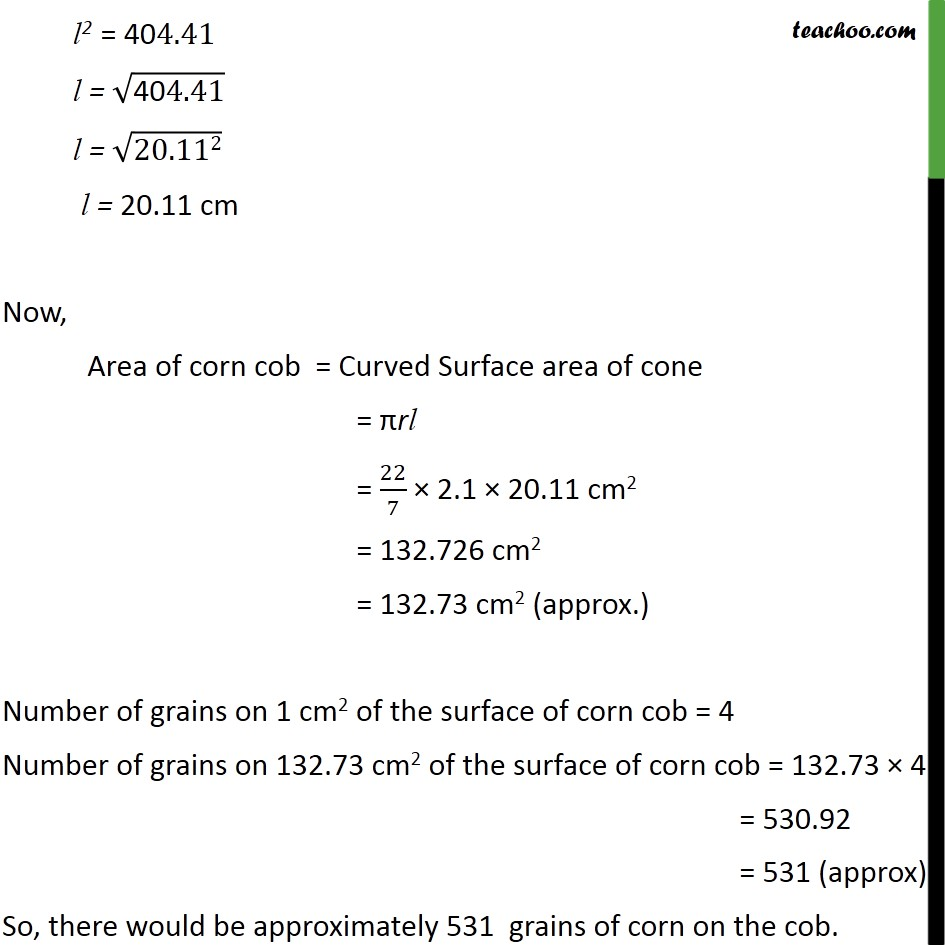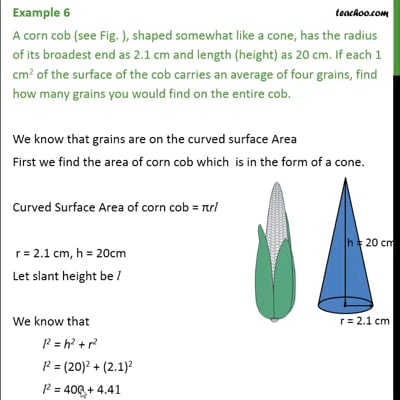Area Of Cone

Chapter 13 Class 9 Surface Areas and Volumes
Concept wiseThis video is only available for Teachoo black users

Introducing your new favourite teacher - Teachoo Black, at only ₹83 per month

### Transcript

Example 6 A corn cob (see Fig. ), shaped somewhat like a cone, has the radius of its broadest end as 2.1 cm and length (height) as 20 cm. If each 1 cm2 of the surface of the cob carries an average of four grains, find how many grains you would find on the entire cob. We know that grains are on the curved surface Area First we find the area of corn cob which is in the form of a cone. Curved Surface Area of corn cob = πrl r = 2.1 cm, h = 20cm Let slant height be l We know that l2 = h2 + r2 l2 = "(20)2 + (2.1)2" l2 = "400 + 4.4" 1 l2 = "40" 4.41 l = √("40" 4.41) l = √("20.112" ) l = 20.11 cm Now, Area of corn cob = Curved Surface area of cone = πrl = 22/7 × 2.1 × 20.11 cm2 = 132.726 cm2 = 132.73 cm2 (approx.) Number of grains on 1 cm2 of the surface of corn cob = 4 Number of grains on 132.73 cm2 of the surface of corn cob = 132.73 × 4 = 530.92 = 531 (approx) So, there would be approximately 531 grains of corn on the cob.